NEET  >  31 Year NEET Previous Year Questions: Thermodynamics - 1

# 31 Year NEET Previous Year Questions: Thermodynamics - 1

Test Description

## 14 Questions MCQ Test Physics Class 11 | 31 Year NEET Previous Year Questions: Thermodynamics - 1

31 Year NEET Previous Year Questions: Thermodynamics - 1 for NEET 2023 is part of Physics Class 11 preparation. The 31 Year NEET Previous Year Questions: Thermodynamics - 1 questions and answers have been prepared according to the NEET exam syllabus.The 31 Year NEET Previous Year Questions: Thermodynamics - 1 MCQs are made for NEET 2023 Exam. Find important definitions, questions, notes, meanings, examples, exercises, MCQs and online tests for 31 Year NEET Previous Year Questions: Thermodynamics - 1 below.
Solutions of 31 Year NEET Previous Year Questions: Thermodynamics - 1 questions in English are available as part of our Physics Class 11 for NEET & 31 Year NEET Previous Year Questions: Thermodynamics - 1 solutions in Hindi for Physics Class 11 course. Download more important topics, notes, lectures and mock test series for NEET Exam by signing up for free. Attempt 31 Year NEET Previous Year Questions: Thermodynamics - 1 | 14 questions in 30 minutes | Mock test for NEET preparation | Free important questions MCQ to study Physics Class 11 for NEET Exam | Download free PDF with solutions
 1 Crore+ students have signed up on EduRev. Have you?
31 Year NEET Previous Year Questions: Thermodynamics - 1 - Question 1

### In thermodynamic processes which of the following statements is not true? 

Detailed Solution for 31 Year NEET Previous Year Questions: Thermodynamics - 1 - Question 1

In an isochoric process volume remains constant whereas pressure remains constant in isobaric process.

31 Year NEET Previous Year Questions: Thermodynamics - 1 - Question 2

### The internal energy change in a system that has absorbed 2 kcals of heat and done 500 J of work is: 

Detailed Solution for 31 Year NEET Previous Year Questions: Thermodynamics - 1 - Question 2

According to first law of thermodynamics
Q = ΔU + W
ΔU = Q – W
= 2 × 4.2 × 1000 – 500 = 8400 –500
= 7900 J

31 Year NEET Previous Year Questions: Thermodynamics - 1 - Question 3

### If ΔU and ΔW represent the increase in internal energy and work done by the system respectively in a thermodynamical process, which of the following is true? 

Detailed Solution for 31 Year NEET Previous Year Questions: Thermodynamics - 1 - Question 3

By first law of thermodynamics,
ΔQ = ΔU + ΔW
In adiabatic process, ΔQ = 0
∴  ΔU = -ΔW
In isothermal process, ΔU = 0
∴  ΔQ -ΔW

31 Year NEET Previous Year Questions: Thermodynamics - 1 - Question 4

During an isothermal expansion, a confined ideal gas does –150 J of work against its surroundings. This implies that 

Detailed Solution for 31 Year NEET Previous Year Questions: Thermodynamics - 1 - Question 4

From the first law of thermodynamics
ΔU = Q + W
For isothermal process, ΔU = 0
Therefore, Q = - W
Given W = - 150
Therefore, Q = + 150
When Q is positive, the heat is added to the gas.

31 Year NEET Previous Year Questions: Thermodynamics - 1 - Question 5

When 1 kg of ice at 0°C melts to water at 0°C, the resulting change in its entropy, taking latent heat of ice to be 80 cal/°C, is 

Detailed Solution for 31 Year NEET Previous Year Questions: Thermodynamics - 1 - Question 5

Change in entropy is given by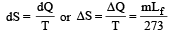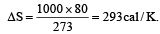31 Year NEET Previous Year Questions: Thermodynamics - 1 - Question 6

A mass of diatomic gas (γ= 1.4) at a pressure of 2 atmospheres is compressed adiabatically so that its temperature rises from 27°C to 927°C.The pressure of the gas in final state is [2011M]

Detailed Solution for 31 Year NEET Previous Year Questions: Thermodynamics - 1 - Question 6

T1 = 273 + 27 = 300K
T2 = 273 + 927 = 1200K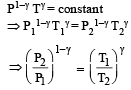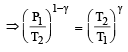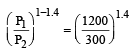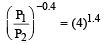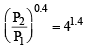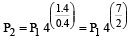= P1 (27) = 2 × 128 = 256 atm

31 Year NEET Previous Year Questions: Thermodynamics - 1 - Question 7

A thermodynamic system is taken through the cycle ABCD as shown in figure. Heat rejected by the gas during the cycle is :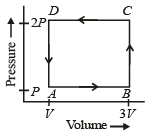Detailed Solution for 31 Year NEET Previous Year Questions: Thermodynamics - 1 - Question 7

∵ Internal energy is the state function.
∴ In cyclie process; ΔU = 0
According to 1st law of thermodynamics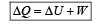So heat absorbed ΔQ = W = Area under the curve
= –  (2V) (P) = –  2PV
So heat rejected  =  2PV

31 Year NEET Previous Year Questions: Thermodynamics - 1 - Question 8

One mole of an ideal gas goes from an initial state A to final state B via two processes : It first undergoes isothermal expansion from volume V to 3V and then its volume is reduced from 3V to V at constant pressure. The correct P-V diagram representing the two processes is : 

Detailed Solution for 31 Year NEET Previous Year Questions: Thermodynamics - 1 - Question 8

1st process is isothermal expansion which is only correct shown in option (d) 2nd process is isobaric compression which is correctly shown in option (d)

31 Year NEET Previous Year Questions: Thermodynamics - 1 - Question 9

If Q1, Q2, Q3 indicate the heat a absorbed by the gas along the three processes and ΔU1, ΔU2, ΔU3 indicate the change in internal energy along the three processes respectively, then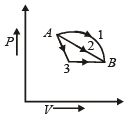Detailed Solution for 31 Year NEET Previous Year Questions: Thermodynamics - 1 - Question 9

Initial and final condition is same for all process ΔU1 =  ΔU2 = ΔU3
from first law of thermodynamics ΔQ = ΔU + ΔW
Work done ΔW1 > ΔW2 > ΔW3 (Area of P.V. graph)
So ΔQ1 > ΔQ2 > ΔQ3

31 Year NEET Previous Year Questions: Thermodynamics - 1 - Question 10

A gas is taken through the cycle A → B → C → A, as shown in figure. What is the net work done by the gas ? [NEET 2013]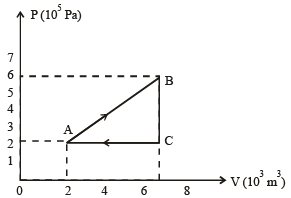Detailed Solution for 31 Year NEET Previous Year Questions: Thermodynamics - 1 - Question 10

Wnet = Area of triangle ABC =1/2(AC × BC)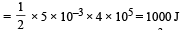31 Year NEET Previous Year Questions: Thermodynamics - 1 - Question 11

During an adiabatic process, the pressure of a gas is found to be proportional to the cube of its temperature. The ratio of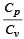for the gas is   [NEET 2013]

Detailed Solution for 31 Year NEET Previous Year Questions: Thermodynamics - 1 - Question 11

According to question P ∝ T3
But as we know for an adiabatic process the pressure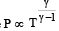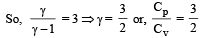31 Year NEET Previous Year Questions: Thermodynamics - 1 - Question 12

A system is taken from state a to state c by two paths adc and abc as shown in the figure. The internal energy at a is Ua = 10 J. Along the path adc the amount of heat absorbed δQ1 = 50 J and the work done δW1 = 20 J whereas along the path abc the heat absorbed δQ2 = 36 J. The amount of work done along the path abc is [NEET Kar. 2013]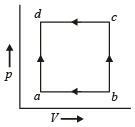Detailed Solution for 31 Year NEET Previous Year Questions: Thermodynamics - 1 - Question 12

From first law of thermodynamics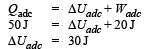Again,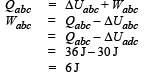31 Year NEET Previous Year Questions: Thermodynamics - 1 - Question 13

Which of the following relations does not give the equation of an adiabatic process, where terms have their usual meaning? [NEET Kar. 2013]

Detailed Solution for 31 Year NEET Previous Year Questions: Thermodynamics - 1 - Question 13

PVγ = constant
TVγ–1 = constant
P1–γTγ = constant.

31 Year NEET Previous Year Questions: Thermodynamics - 1 - Question 14

Two Carnot engines A and B are operated in series. The engine A receives heat from the source at temperature T1 and rejects the heat to the sink at temperature T. The second engine B receives the heat at temperature T and rejects to its sink at temperature T2. For what value of T the efficiencies of the two engines are equal? [NEET Kar. 2013]

Detailed Solution for 31 Year NEET Previous Year Questions: Thermodynamics - 1 - Question 14

Efficiency of engine A,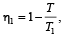Efficiency of engine B,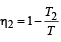Here, η1 = η2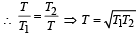## Physics Class 11

127 videos|464 docs|210 tests
 Use Code STAYHOME200 and get INR 200 additional OFF Use Coupon Code
Information about 31 Year NEET Previous Year Questions: Thermodynamics - 1 Page
In this test you can find the Exam questions for 31 Year NEET Previous Year Questions: Thermodynamics - 1 solved & explained in the simplest way possible. Besides giving Questions and answers for 31 Year NEET Previous Year Questions: Thermodynamics - 1, EduRev gives you an ample number of Online tests for practice

## Physics Class 11

127 videos|464 docs|210 tests

### How to Prepare for NEET

Read our guide to prepare for NEET which is created by Toppers & the best Teachers